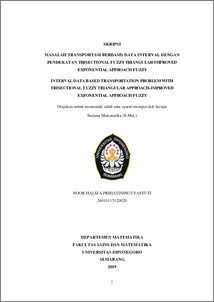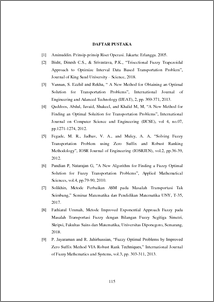# INTERVAL DATA BASED TRANSPORTATION PROBLEM WITH TRISECTIONAL FUZZY TRIANGULAR APPROACH-IMPROVED EXPONENTIAL APPROACH FUZZY

Prihatiningtyastuti, Noor Haliza (2019) INTERVAL DATA BASED TRANSPORTATION PROBLEM WITH TRISECTIONAL FUZZY TRIANGULAR APPROACH-IMPROVED EXPONENTIAL APPROACH FUZZY. Undergraduate thesis, UNDIP.Preview
PDF
55KbPDF
Restricted to Repository staff only

1066KbPreview
PDF
171Kb

## Abstract

Interval data based transportation problem (IBTP) is a form of transportation problem where data is provided in the form of interval. There are several alternatives or methods that have been available in solving the interval data based transportation problem. One of alternative is to change the interval-based transportation problem into the form of a fuzzy transportation problem. The parameter used in the fuzzy transportation problem is the symmetry triangular fuzzy number. In this Final Project, a Trisectional Fuzzy Triangular approach is used to represent the interval data based transportation problem in the form of a fuzzy transportation problem. To solve the interval-based transportation problem, the Improved Exponential Approach Fuzzy method is applied. Trisectional Fuzzy Triangular Approach-Improved Exponential Approach Fuzzy provides an optimal solution to the interval data based transportation problem. Keyword: Interval, Trisectional Triangular Fuzzy Approach, Improved Exponential Approach Fuzzy, symmetry triangular fuzzy number, Graded Mean Ranking.

Item Type: Thesis (Undergraduate) Q Science > QA Mathematics Faculty of Science and Mathematics > Department of Mathematics 84267 INVALID USER 13 Jun 2022 11:06 13 Jun 2022 11:06

Repository Staff Only: item control page